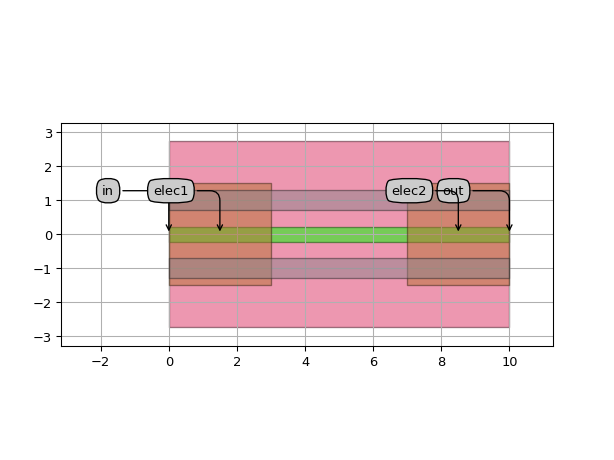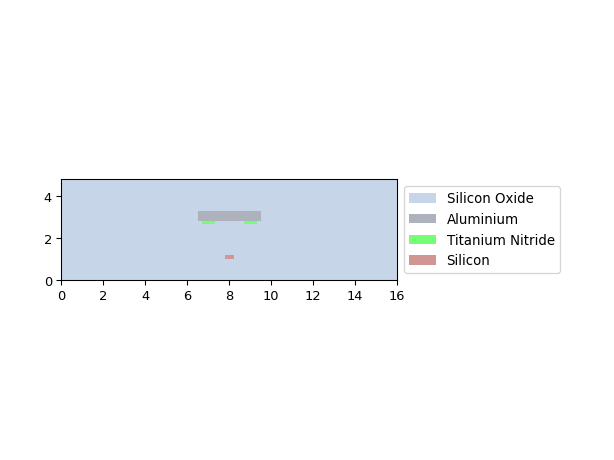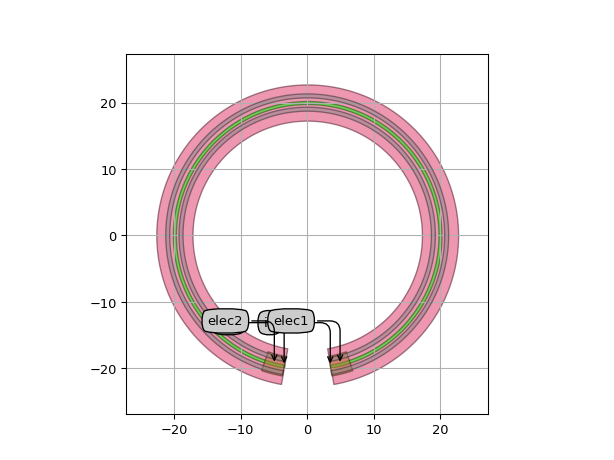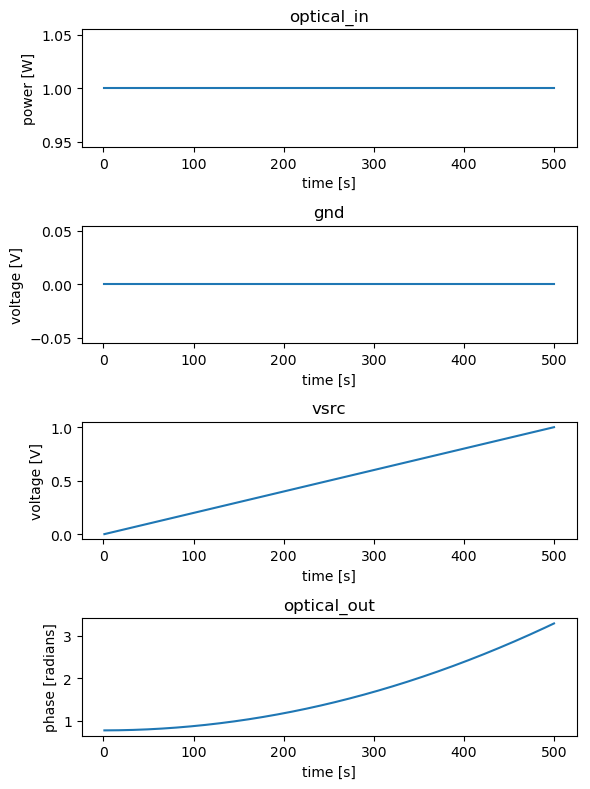# Heaters¶

SiFab contains one heater:

## HeatedWaveguide¶

This component consists of a waveguide with a heater layer on each side, drawn on the HT layer. It is used to change the phase of the light travelling through the waveguide by exploiting the thermo-optic effect in silicon. At the beginning and at the end of the waveguide, metal vias are used to connect the heater metal to a metal contact pad on the M1 layer.

The following parameters can be changed:

• heater_width: Width of the heater layer
• heater_offset: Offset of the heaters from the center
• m1_width: Width of the M1 contact area
• m1_length: Length of the M1 contact areas

Reference

Click on the name of the component below to see the complete PCell reference.

 si_fab.all.HeatedWaveguide Phase shifter waveguide with heater layers on each side.

Example

import si_fab.all as pdk
from ipkiss3 import all as i3

# Heater with straight shape
ht = pdk.HeatedWaveguide(
heater_width=0.6,
heater_offset=1.0,
m1_width=1.0,
m1_length=3.0,
)
ht_lv = ht.Layout(shape=[(0.0, 0.0), (10.0, 0.0)])
ht_lv.visualize(annotate=True)

xs = ht_lv.cross_section(cross_section_path=i3.Shape([(1.0, -8.0), (1.0, 8.0)]))
xs.visualize()

# Heater with rounded shape
ht = pdk.HeatedWaveguide()
ht_lv = ht.Layout(shape=ht_shape)
ht_lv.visualize(annotate=True)### Model and simulation recipes¶

The phase of the waveguide is calculated using the effective index ($$n_{eff}$$) of the trace template (trace_template) used to draw the waveguide. The model of the heater adds a phase modulation to the phase of the waveguide that is calculated as follows:

$\Delta \Phi = \frac{\pi V_d^2}{P_{\pi\square}}\frac{W}{L}$

where

• $$L$$ and $$W$$ are the length and width of the heater, respectively;
• $$V_d$$ is the voltage drop over the phase shifter length;
• $$P_{\pi\square}$$ is the power required on a square of heater material with a unit sheet resistance (1 $$\Omega / \square$$) to achieve a $$\pi$$ phase shift.

Usign the power dissipated over the heater to achieve a $$\pi$$ phase shift can be calculated as:

$P_{\pi} = \frac{P_{\pi\square}}{R_{sh}}$

where $$R_{sh}$$ is the sheet resistance of the heater metal.

Using the simulation recipe simulate_heater one can easily check the phase shift caused by a voltage sweep from $$0$$ to $$1$$, as well as the associated current density. The sheet resistance $$R_{sh} = 0.5$$ $$\Omega / \square$$ is stored in the SiFab PDK and can be considered fixed.

Reference

Click on the name of the function below to see the complete API reference of the simulation recipe.

 si_fab.components.heater.simulation.simulate.simulate_heater Simulation recipe to simulate a heater by sweeping the bias voltage.

Example

import si_fab.all as pdk
from si_fab.components.heater.simulation.simulate import simulate_heater
from si_fab.components.heater.pcell.cell import r_sheet
import pylab as plt
import numpy as np
import os

# Heated waveguide
name = "heater_sweep"
length = 100
ht = pdk.HeatedWaveguide(
heater_width=0.6,
heater_offset=1.0,
m1_width=1.0,
m1_length=3.0,
)
ht_lv = ht.Layout(shape=[(0.0, 0.0), (length, 0.0)])

# Power for pi phase shift per square of unit sheet resistance
p_pi = 30e-3  # W
p_pi_sq = r_sheet * p_pi
ht.CircuitModel(p_pi_sq=p_pi_sq)

results = simulate_heater(
cell=ht,
v_start=0,
v_end=1,
nsteps=500,
center_wavelength=1.5,
debug=False,
)
times = results.timesteps

def phase_unwrap_normalize(transmission):
unwrapped = np.unwrap(np.angle(transmission))
return unwrapped - unwrapped

signals = ["optical_in", "gnd", "vsrc", "optical_out"]
process = [np.real, np.real, np.real, phase_unwrap_normalize]
ylabels = ["power [W]", "voltage [V]", "voltage [V]", "phase [radians]"]

fig, axs = plt.subplots(nrows=len(signals), ncols=1, figsize=(6, 8))
for axes, process, signal, ylabel in zip(axs, process, signals, ylabels):
data = process(results[signal])
axes.set_title(signal)
axes.plot(times[1:], data[1:])
axes.set_ylabel(ylabel)
axes.set_xlabel("time [s]")
plt.tight_layout()
fig.savefig(os.path.join("{}.png".format(name)), bbox_inches='tight')
plt.show()